# Equivalent Fractions BINGO

Equivalent Fractions BINGO is a fun educational game for children to practice equivalent fractions. The game has two modes: Easy & Challenge. Easy mode of play displays a pie graphic to represent the fraction. The denominator is represented by the total number of slices and the numerator is represented by the colored slices. Challenge mode displays only the numerical fraction at first. If the student needs help it will introduce a pie graphic representing only the denominator. If they need further help it will display the shaded slices representing the numerator. Challenge points are awarded based on how much help the student needed to correctly answer the question. Less help = more points!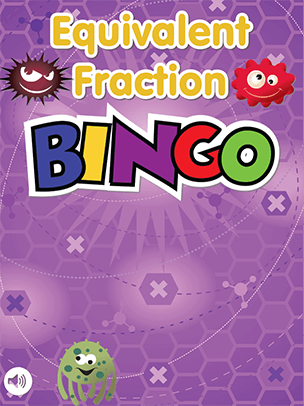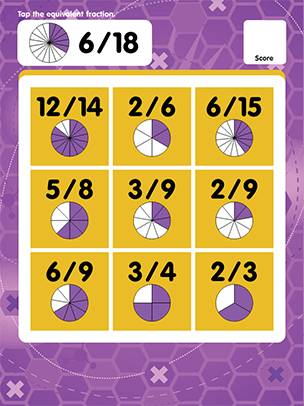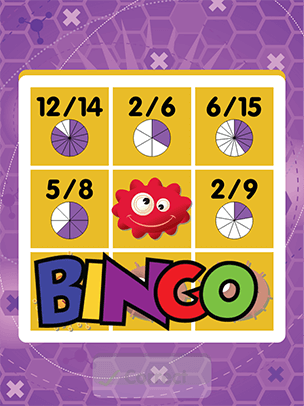Equivalent Fractions BINGO is a fun educational game for children to practice equivalent fractions. The game has two modes: Easy & Challenge. Easy mode of play displays a pie graphic to represent the fraction. The denominator is represented by the total number of slices and the numerator is represented by the colored slices. Challenge mode displays only the numerical fraction at first. If the student needs help it will introduce a pie graphic representing only the denominator. If they need further help it will display the shaded slices representing the numerator. Challenge points are awarded based on how much help the student needed to correctly answer the question. Less help = more points!

# Equivalent Fractions BINGO

Equivalent Fractions BINGO is a fun educational game for children to practice equivalent fractions. The game has two modes: Easy & Challenge. Easy mode of play displays a pie graphic to represent the fraction. The denominator is represented by the total number of slices and the numerator is represented by the colored slices. Challenge mode displays only the numerical fraction at first. If the student needs help it will introduce a pie graphic representing only the denominator. If they need further help it will display the shaded slices representing the numerator. Challenge points are awarded based on how much help the student needed to correctly answer the question. Less help = more points!

Equivalent Fractions BINGO is a fun educational game for children to practice equivalent fractions. The game has two modes: Easy & Challenge. Easy mode of play displays a pie graphic to represent the fraction. The denominator is represented by the total number of slices and the numerator is represented by the colored slices. Challenge mode displays only the numerical fraction at first. If the student needs help it will introduce a pie graphic representing only the denominator. If they need further help it will display the shaded slices representing the numerator. Challenge points are awarded based on how much help the student needed to correctly answer the question. Less help = more points!Equivalent Fractions BINGO is a fun educational game for children to practice equivalent fractions. The game has two modes: Easy & Challenge. Easy mode of play displays a pie graphic to represent the fraction. The denominator is represented by the total number of slices and the numerator is represented by the colored slices. Challenge mode displays only the numerical fraction at first. If the student needs help it will introduce a pie graphic representing only the denominator. If they need further help it will display the shaded slices representing the numerator. Challenge points are awarded based on how much help the student needed to correctly answer the question. Less help = more points!Equivalent Fractions BINGO is a fun educational game for children to practice equivalent fractions. The game has two modes: Easy & Challenge. Easy mode of play displays a pie graphic to represent the fraction. The denominator is represented by the total number of slices and the numerator is represented by the colored slices. Challenge mode displays only the numerical fraction at first. If the student needs help it will introduce a pie graphic representing only the denominator. If they need further help it will display the shaded slices representing the numerator. Challenge points are awarded based on how much help the student needed to correctly answer the question. Less help = more points!

# Equivalent Fractions BINGO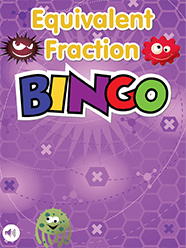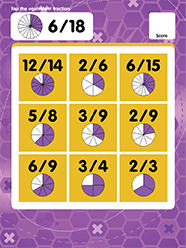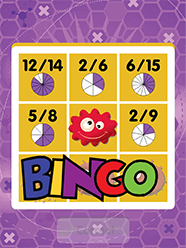Equivalent Fractions BINGO is a fun educational game for children to practice equivalent fractions. The game has two modes: Easy & Challenge. Easy mode of play displays a pie graphic to represent the fraction. The denominator is represented by the total number of slices and the numerator is represented by the colored slices. Challenge mode displays only the numerical fraction at first. If the student needs help it will introduce a pie graphic representing only the denominator. If they need further help it will display the shaded slices representing the numerator. Challenge points are awarded based on how much help the student needed to correctly answer the question. Less help = more points!